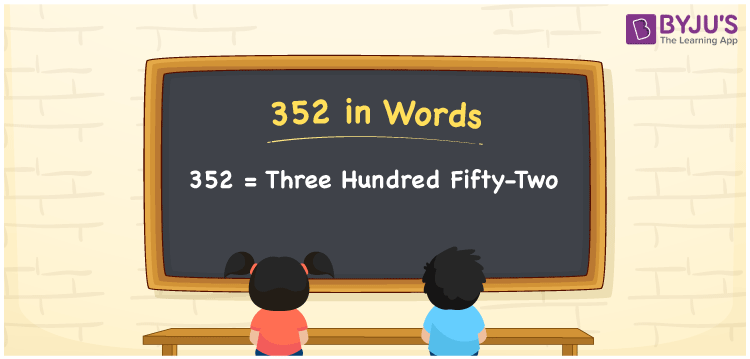# 352 in Words

352 in words is written as Three hundred fifty-two. In both the International System of Numerals and the Indian System of Numerals, 352 is written as Three hundred fifty-two. The number 352 is a Cardinal Number as it represents some quantity. For example, “there are 352 chairs in the room”.

 352 in Words Three hundred fifty-two Three hundred fifty-two in Number 352

## 352 in English Words

We write 352 in English Words using the letters of the English alphabet. Therefore, we read 352 in English as “Three hundred fifty-two.”## How to Write 352 in Words?

To write 352 in words, we shall use the place value chart. In the place value chart, write 3 in the hundreds, 5 in the tens, and 2 in the ones, respectively. Now let us make a place value chart to write the number 352 in words.

 Hundreds Tens Ones 3 5 2

Thus, we can write the expanded form as

3 × Hundred + 5 × Ten + 2× One

= 3 × 100 + 5 × 10 + 2 × 1

= 300 + 50 + 2

= 352

= Three hundred fifty-two.

352 is a natural number, the successor of 351 and the predecessor of 353.

352 in words – Three hundred fifty-two

• Is 352 an odd number? – No
• Is 352 an even number? – Yes
• Is 352 a perfect square number? – No
• Is 352 a perfect cube number? – No
• Is 352 a prime number? – No
• Is 352 a composite number? – Yes

## Frequently Asked Questions on 352 in Words

Q1

### How to write 352 in words?

352 in words is written as Three hundred fifty-two.
Q2

### How to write 352 in the International and Indian System of Numerals?

In both, the system of numerals, 352 in words, is written as Three hundred fifty-two.
Q3

### What is the preceding number of 352?

The number that precedes 352 is 351.